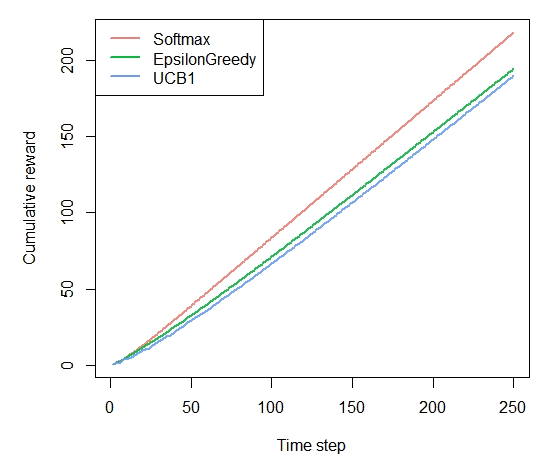# Demo: Replication of John Myles White, Bandit Algorithms for Website Optimization

#### 2020-07-25

Simulation of multi-armed Bandit policies following John Myles White’s “Bandit algorithms for website_optimization”.

The book, which offers a comprehensive entry-level introduction to context-free bandit policies, is available here: John Myles White. The orignal (Python) code for the book can be found in its complementary GitHub repository.

## Discrepancies with the book

We did encounter one minor issue with the original code used to generate the plots in the book: its bandit policies always choose the first arm (in other words, the arm with the lowest index) when rewards between arms are tied. In the original Python code:

def ind_max(x):
m = max(x)
return x.index(m)

This introduces a bias that adds up over time, changing simulations’ results and plots. In R, (which uses 1 based indexing) this corresponds to always doing:

rewards      <- c(0,0,1,0,1)
max_reward   <-which.max(rewards)
max_reward   # always contains lowest index, 3 in this case, biasing results

Contextual correctly returns the index of one of the tied maxima random, fixing the bias, resulting in slightly different plots. In Python, a patch along the following lines ought to resolve this issue:

def ind_max(x):
max_value = max(x)
max_keys = [k for k, v in enumerate(x) if v == max_value]
return random.choice(max_keys)

In R, this corresponds to:

rewards      <- c(0,0,1,0,1)
max_reward   <- which(rewards == max(rewards))
if(length(max_reward) > 1){
max_reward <- sample(max_reward, 1)
}
max_reward   # randomly assigned either 3 or 5, no bias

To illustrate, a replication of the original Figure 4-3 when running a simulation with the biased arm selection, (as used in the book) on the left, contextual’s unbiased simulation to the right: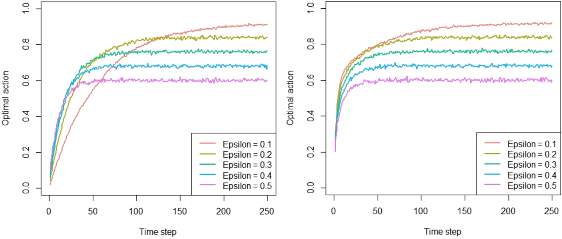## Chapter 4 - Debugging and epsilon greedy

prob_per_arm       <- c(0.1, 0.1, 0.1, 0.1, 0.9)
horizon            <- 250
simulations        <- 5000

bandit             <- BasicBernoulliBandit$new(prob_per_arm) agents <- list(Agent$new(EpsilonGreedyPolicy$new(0.1), bandit, "Epsilon = 0.1"), Agent$new(EpsilonGreedyPolicy$new(0.2), bandit, "Epsilon = 0.2"), Agent$new(EpsilonGreedyPolicy$new(0.3), bandit, "Epsilon = 0.3"), Agent$new(EpsilonGreedyPolicy$new(0.4), bandit, "Epsilon = 0.4"), Agent$new(EpsilonGreedyPolicy$new(0.5), bandit, "Epsilon = 0.5")) simulation <- Simulator$new(agents, horizon, simulations)
history            <- simulation$run() ### Figure 5-2. How often does the softmax algorithm select the best arm? plot(history, type = "optimal", legend_position = "bottomright", ylim = c(0,1))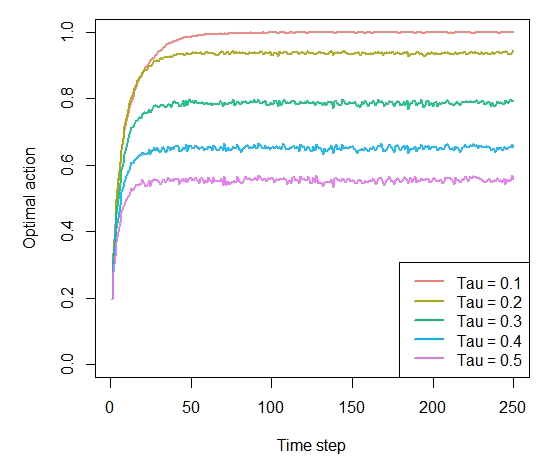### Figure 5-3. How much reward does the softmax algorithm earn on average? plot(history, type = "average", regret = FALSE, legend_position = "bottomright", ylim = c(0,1))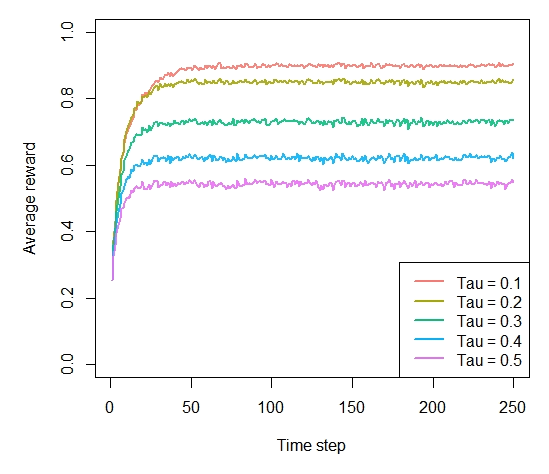### Figure 5-4. How much reward has the softmax algorithm earned by trial t? plot(history, type = "cumulative", regret = FALSE)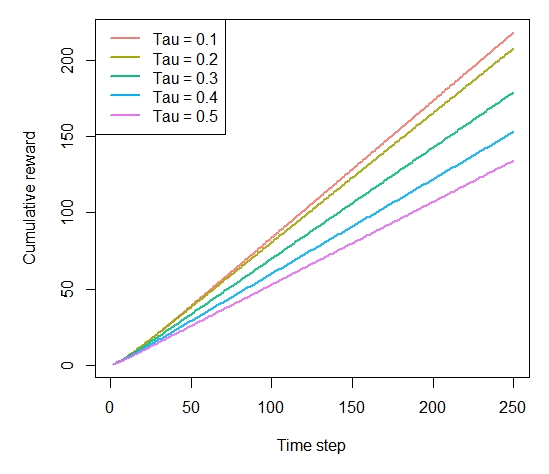## Chapter 6 - UCB agents <- list(Agent$new(SoftmaxPolicy$new(0.1), bandit, "Softmax"), Agent$new(EpsilonGreedyPolicy$new(0.1), bandit, "EpsilonGreedy"), Agent$new(UCB1Policy$new(), bandit, "UCB1")) simulation <- Simulator$new(agents, horizon, simulations)
history            <- simulation\$run()

### Figure 6-3. How often does the UCB algorithm select the best arm?

plot(history, type = "optimal", legend_position = "bottomright", ylim = c(0,1))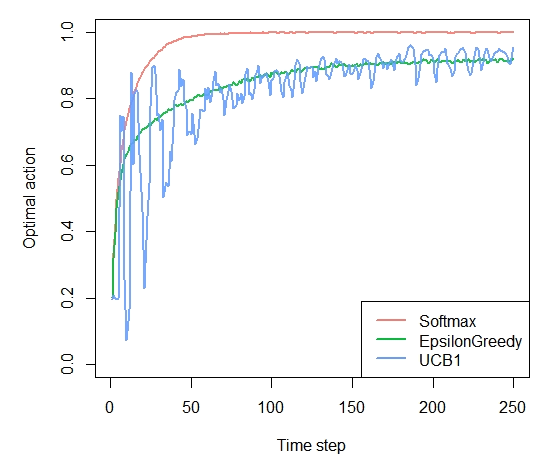### Figure 6-4. How much reward does the UCB algorithm earn on average?

plot(history, type = "average", regret = FALSE, legend_position = "bottomright", ylim = c(0,1))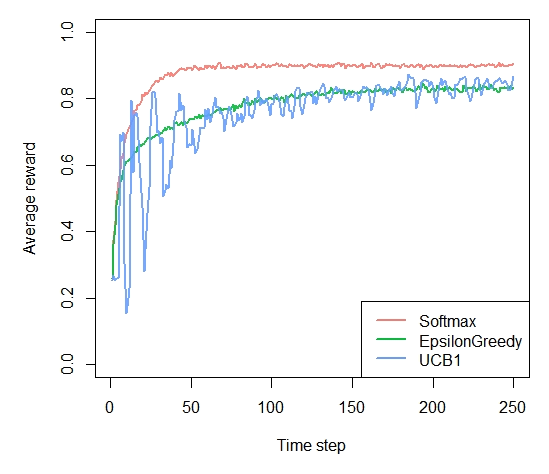### Figure 6-5. How much reward has the UCB algorithm earned by trial t?

plot(history, type = "cumulative", regret = FALSE)